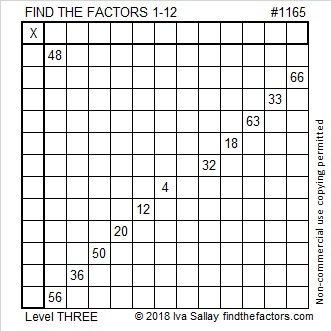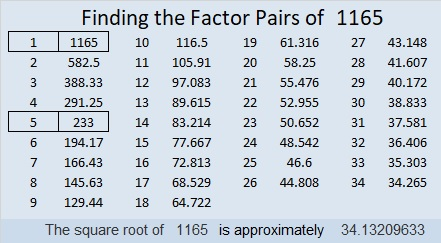1165 and Level 3

Just because you start with the clues at the top of the puzzle and work down cell by cell to solve a level 3 puzzle doesn’t mean that you won’t have to do any thinking. Believe me, you will still have to THINK to solve this puzzle!Print the puzzles or type the solution in this excel file: 12 factors 1161-1173

Now I’ll write a little bit about the number 1165:

• 1165 is a composite number.
• Prime factorization: 1165 = 5 × 233
• The exponents in the prime factorization are 1 and 1. Adding one to each and multiplying we get (1 + 1)(1 + 1) = 2 × 2 = 4. Therefore 1165 has exactly 4 factors.
• Factors of 1165: 1, 5, 233, 1165
• Factor pairs: 1165 = 1 × 1165 or 5 × 233
• 1165 has no square factors that allow its square root to be simplified. √1165 ≈ 34.13209634² + 3² = 1165
29² + 18² = 1165

1165 is the hypotenuse of FOUR Pythagorean triples:
204-1147-1165 calculated from 2(34)(3), 34² – 3², 34² + 3²
517-1044-1165 calculated from 29² – 18², 2(29)(18), 29² + 18²
525-1040-1165 which is 5 times (105-208-233)
699-932-1165 which is (3-4-5) times 233